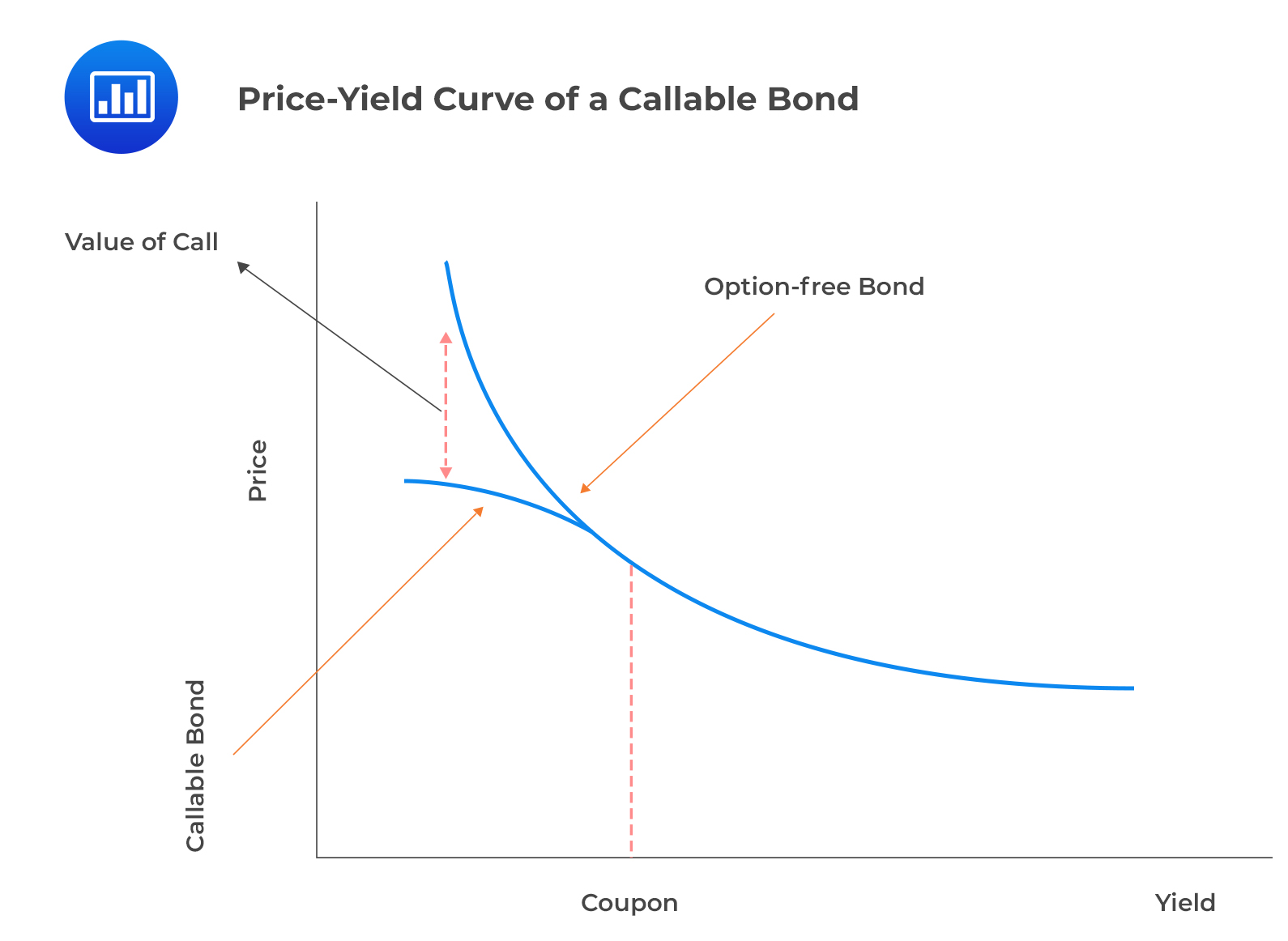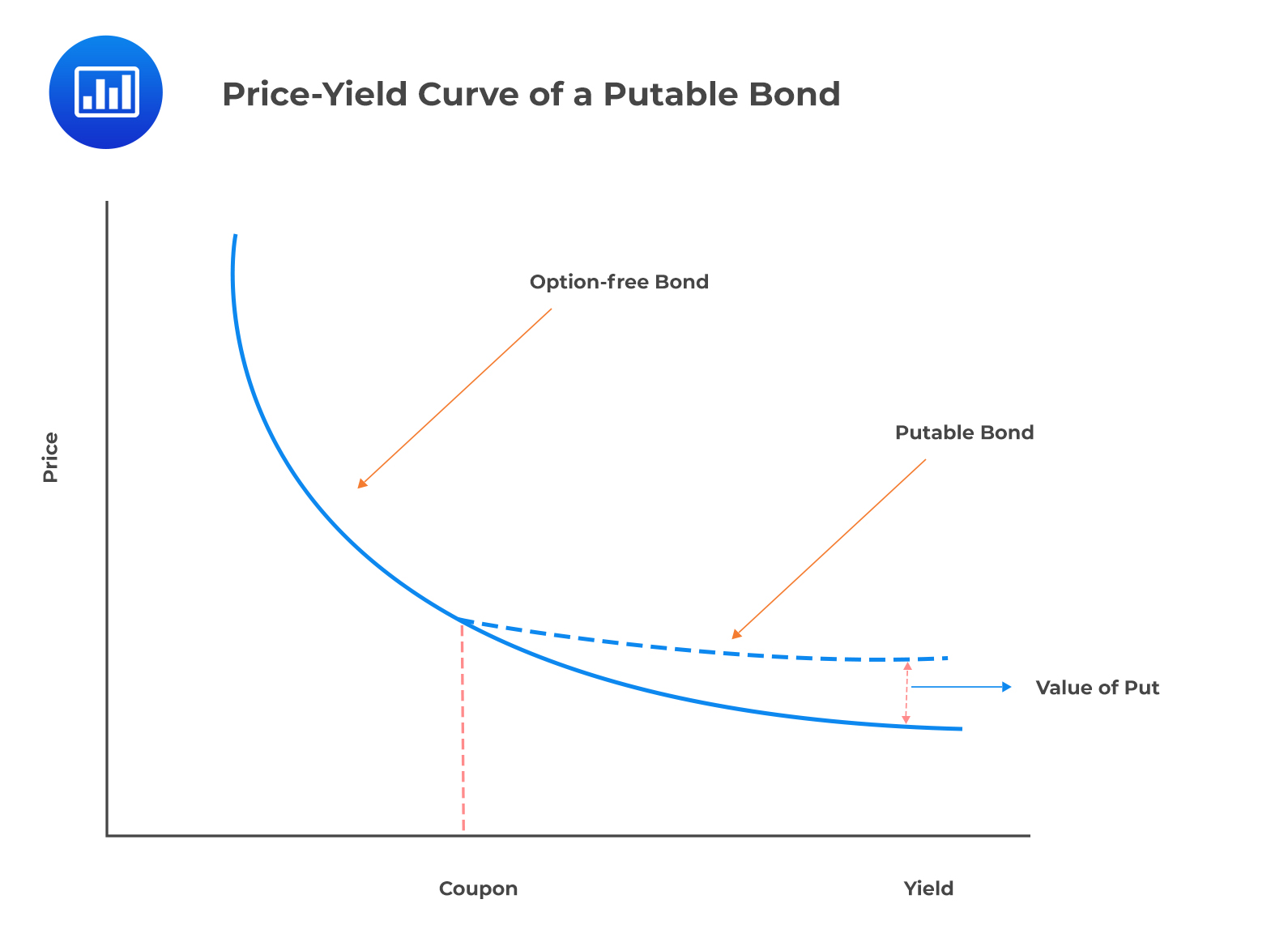# Valuing Embedded Options

According to the arbitrage-free framework, the value of a bond with embedded options is the sum of the arbitrage-free value of the option-free bond (straight bond) and the arbitrage-free values of any embedded options.

## Embedded Calls

A callable bond is similar to a portfolio with a long position on a straight bond and a short position on a call option. The issuer makes the decision to exercise the call option.

\begin{align*} \text{Value of callable bond}& =\text{Value of straight bond} \\ & –\text{Value of issuer call option} \\ \text{Value of issuer call option}& =\text{Value of straight bond} \\ & – \text{Value of callable bond} \\ \end{align*}

i.e.,

\begin{align*} V_{\text{Callable}} &= V_{\text{Straight}} – V_{\text{Call}} \\ V_{\text{Call}} &= V_{\text{Straight}} – V_{\text{Callable}} \end{align*}

This has been illustrated in the following diagram:It is worth noting that callable bonds are riskier, have higher spreads, and generate higher yields relative to put bonds.

## Embedded Puts

The investor has a long position in both the straight bond and the put option.

\begin{align*} \text{Value of putable bond} & =\text{Value of straight bond} \\ & +\text{Value of investor put option} \\ \text{Value of investor put option} & =\text{Value of putable bond} \\ & – \text{Value of straight bond} \end{align*}

i.e.,

\begin{align*} V_{\text{Putable}}= V_{\text{Straight}}+ V_{\text{Put}} \\ V_{\text{Put}}=V_{\text{Putable}} – V_{\text{Straight}} \end{align*}

The concept of embedded puts is illustrated in the following diagram:## Question

The value of an option free, 5% annual coupon bond that matures in three years is $106.80. If the value of a callable bond with similar terms is$105.50, the value of the issuer call option is closest to:

1. -$1.30. 2.$0.00.
3. \$1.30.

#### Solution

\begin{align*} \text{Value of issuer call option} &=\text{Value of straight bond} \\ & – \text{Value of callable bond} \\ V_{\text{Callable}} &=105.50 \\ V_{\text{Straight}} &=106.80 \\ V_{\text{Call}} &=106.80-105.50=1.30 \end{align*}

Reading 30: Valuation and Analysis of Bonds with Embedded Options

LOS 30 (b) Explain the relationships between the values of a callable or putable bond, the underlying option free (straight) bond, and the embedded option.

Shop CFA® Exam Prep

Offered by AnalystPrepLevel I
Level II
Level III
All Three Levels
Featured Shop FRM® Exam PrepFRM Part I
FRM Part II
FRM Part I & Part II
Learn with Us

Subscribe to our newsletter and keep up with the latest and greatest tips for success
Shop Actuarial Exams PrepExam P (Probability)
Exam FM (Financial Mathematics)
Exams P & FMGMAT® Complete CourseDaniel Glyn
2021-03-24
I have finished my FRM1 thanks to AnalystPrep. And now using AnalystPrep for my FRM2 preparation. Professor Forjan is brilliant. He gives such good explanations and analogies. And more than anything makes learning fun. A big thank you to Analystprep and Professor Forjan. 5 stars all the way!michael walshe
2021-03-18
Professor James' videos are excellent for understanding the underlying theories behind financial engineering / financial analysis. The AnalystPrep videos were better than any of the others that I searched through on YouTube for providing a clear explanation of some concepts, such as Portfolio theory, CAPM, and Arbitrage Pricing theory. Watching these cleared up many of the unclarities I had in my head. Highly recommended.Nyka Smith
2021-02-18
Every concept is very well explained by Nilay Arun. kudos to you man!2021-02-13